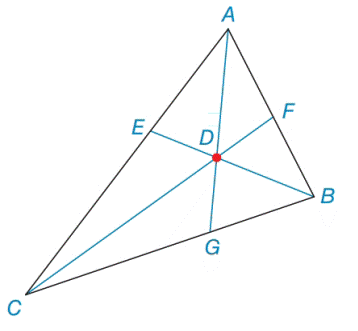Chapter 7.2, Problem 39EElementary Geometry For College St...

7th Edition
Alexander + 2 others
ISBN: 9781337614085

Solutions

Chapter
SectionElementary Geometry For College St...

7th Edition
Alexander + 2 others
ISBN: 9781337614085
Textbook Problem

In Δ A B C , the bisectors of the angle are concurrent at point D . If m ∠ C A B = 64 ∘ and m ∠ A B C = 82 ∘ , find m ∠ E D C .Exercises 39, 40

To determine

To find:

The angle mEDC in triangle ABC.

Explanation

Definition:

Angle bisector of a triangle:

The angle bisector of a triangle is a line segment that bisects one of the vertex angles of a triangle.

In general an angle bisector is equidistant from the sides of the angle when measured along a segment perpendicular to the sides of the angle.

Given:

In ΔABC, the bisectors of the angle are concurrent at point D. If mCAB=64 and mABC=82.

Clarification:

The sum of measures of three angles of any triangle is equal to 180.

By the given triangle ABC,

mCAB+mABC+mBCA=18064+82+mBCA=180Subtracting64and82onbothsides,64+82+mBCA6482=1806482mBCA=34

Next we consider the triangle ECB,

In above figure BE bisects the B vertex angles of a triangle.

And also mBCA=mECB=34.

mECB+mCBE+mBEC=18034+B2+mBEC=18034+822+mBEC=18034+41+mBEC=180Subtracting34and41onbothsides,

Still sussing out bartleby?

Check out a sample textbook solution.

See a sample solution

The Solution to Your Study Problems

Bartleby provides explanations to thousands of textbook problems written by our experts, many with advanced degrees!

Get Started

In Exercises 73-80, find the indicated limits, if they exist. 75. limx3x3+x2+1x3+1

Applied Calculus for the Managerial, Life, and Social Sciences: A Brief Approach

Evaluate the indefinite integral. x(2x+5)8dx

Single Variable Calculus: Early Transcendentals, Volume I

Find the mean for the scores in the following frequency distribution table: X f 6 1 5 4 4 2 3 2 2 1

Essentials of Statistics for The Behavioral Sciences (MindTap Course List)

Change 60 mi/h to ft/s.

Elementary Technical Mathematics

Solve the differential equation. 6. dxdt=1t+xtx

Single Variable Calculus: Early Transcendentals

True or False: If f(c) exists and f has a local minimum at c, then f(c) = 0.

Study Guide for Stewart's Single Variable Calculus: Early Transcendentals, 8th

The equation of the tangent plane to for is:

Study Guide for Stewart's Multivariable Calculus, 8th